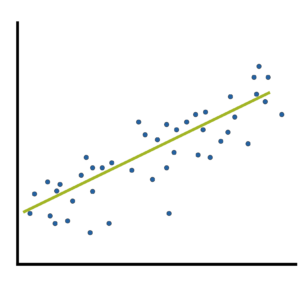# effect size statistics

### Two Types of Effect Size Statistic: Standardized and Unstandardized

June 26th, 2023 by

Effect size statistics are all the rage these days.

Journal editors are demanding them. Committees won’t pass dissertations without them.But the reason to compute them is not just that someone wants them — they can truly help you understand your data analysis.

### What Is an Effect Size Statistic?When many of us hear “Effect Size Statistic,” we immediately think we need one of a few statistics: Eta-squared, Cohen’s d, R-squared.
And yes, these definitely qualify. But the concept of an effect size statistic is actually much broader. Here’s a description from a nice article on effect size statistics:

“… information about the magnitude and direction of the difference between two groups or the relationship between two variables.

– Joseph A. Durlak, “How to Select, Calculate, and Interpret Effect Sizes”

If you think about it, many familiar statistics fit this description. Regression coefficients give information about the magnitude and direction of the relationship between two variables. So do correlation coefficients. (more…)

### Odds Ratio: Standardized or Unstandardized Effect Size?

September 7th, 2021 by

Effect size statistics are extremely important for interpreting statistical results. The emphasis on reporting them has been a great development over the past decade. (more…)

### Effect Size Statistics: How to Calculate the Odds Ratio from a Chi-Square Cross-tabulation Table

August 12th, 2020 by

Lest you believe that odds ratios are merely the domain of logistic regression, I’m here to tell you it’s not true.

One of the simplest ways to calculate an odds ratio is from a cross tabulation table.

We usually analyze these tables with a categorical statistical test. There are a few options, depending on the sample size and the design, but common ones are Chi-Square test of independence or homogeneity, or a Fisher’s exact test.

### Member Training: Interpretation of Effect Size Statistics

August 30th, 2019 byEffect size statistics are required by most journals and committees these days ⁠— for good reason.

They communicate just how big the effects are in your statistical results ⁠— something p-values can’t do.

But they’re only useful if you can choose the most appropriate one and if you can interpret it.

This can be hard in even simple statistical tests. But once you get into  complicated models, it’s a whole new story. (more…)

### Member Training: An Overview of Effect Size Statistics and Why They are So Important

July 1st, 2015 by

Whenever we run an analysis of variance or run a regression one of the first things we do is look at the p-value of our predictor variables to determine whetherthey are statistically significant. When the variable is statistically significant, did you ever stop and ask yourself how significant it is? (more…)

### Effect Size Statistics in Logistic Regression

May 18th, 2015 by

Effect size statistics are expected by many journal editors these days.

If you’re running an ANOVA, t-test, or linear regression model, it’s pretty straightforward which ones to report.

Things get trickier, though, once you venture into other types of models.` (more…)`